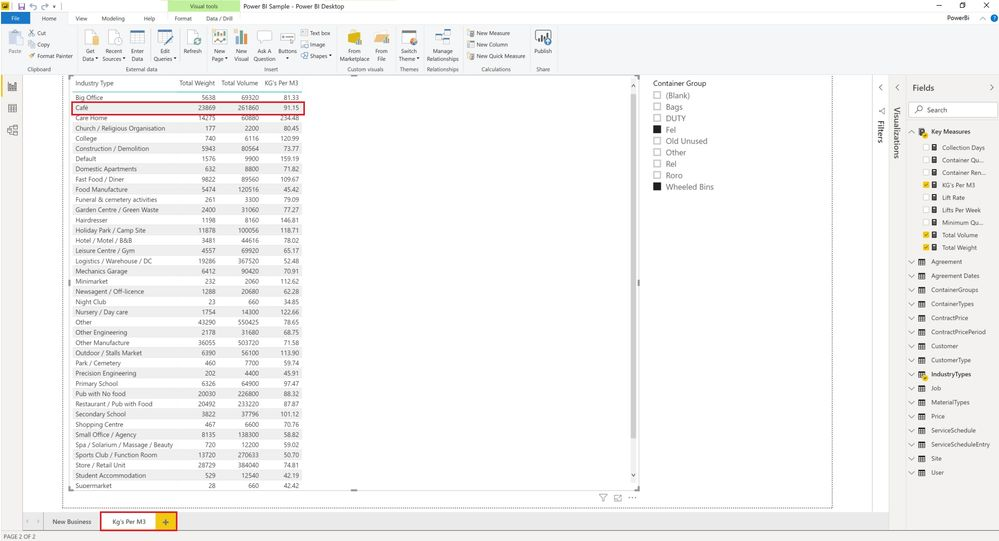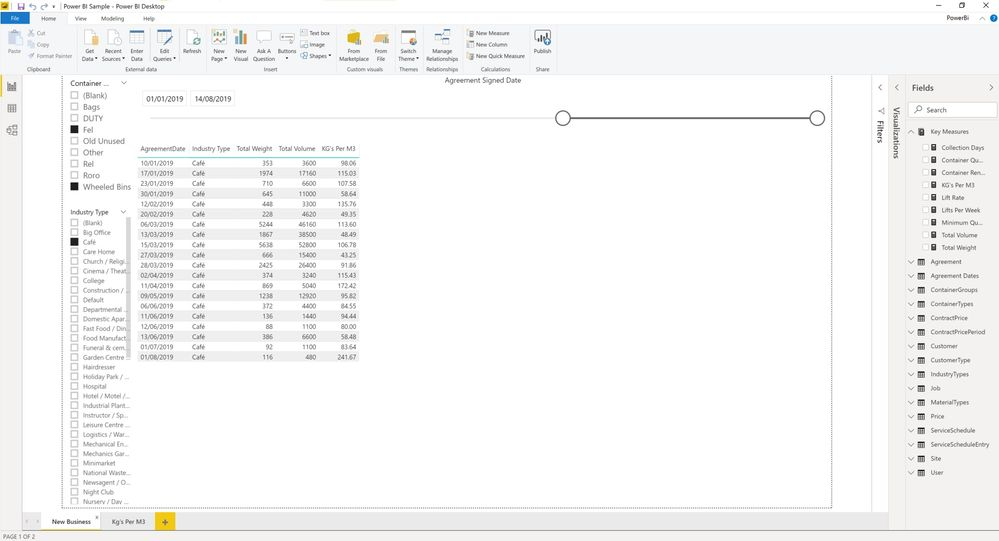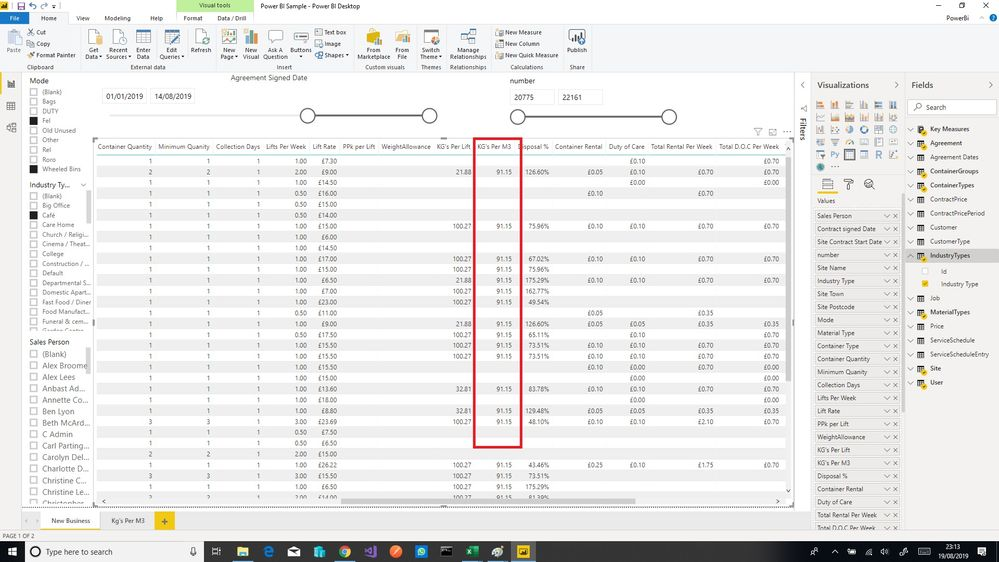cancel
Showing results for
Did you mean:Helper I

## DAX calculation in a different context

Hi,

Im trying to calculate Kg's per M3 for industry types based on all the jobs we have already done and then use this value in a report in a different context. i'e' in the context of a service agreement. The problem is because the context has changed it doing the calculations against jobs of the particular agreements instead of all the jobs which is what I want.

For eaxmple if i take Cafe as an example, the weight, volume and kg's per m3 is calculations are correct.If I use this in a different report and add on agreements I get the following:What I want to achieve is below, how can I do this?https://www.dropbox.com/s/tfiu1tnlsessn47/Power%20BI%20Sample.pbix?dl=0

Nathan

1 ACCEPTED SOLUTIONSuper User

```KG's Per M3 =
IF ( NOT ISBLANK ( [Total Weight] ),
CALCULATE(
DIVIDE([Total Weight],
DIVIDE([Total Volume],1000))
,ALLSELECTED(Agreement)
)
)```6 REPLIES 6Super User

```KG's Per M3 =
IF ( NOT ISBLANK ( [Total Weight] ),
CALCULATE(
DIVIDE([Total Weight],
DIVIDE([Total Volume],1000))
,ALLSELECTED(Agreement)
)
)```Helper I

I've updated the report but I'm getting gaps where there should be the same value, is this due to the other tables in my report?

https://www.dropbox.com/s/4gizjerw7ddd23k/Power%20BI%20Sample.pbix?dl=0

NathanMany Thanks NathanSuper User

Because the [KG's Per M3] measure needs [Total Weight] and [Total Volume] and on those line with blanks there is no weight or volume we get blank.  What would be the basis of the calculation for those lines?  Meaning, what should it be and how did you get to that number?Helper I

The KG's Per M3 is supposed to be based on all the jobs of an industry type, for Cafe is think it was 91.15. so as long as the agreement is of type Cafe I want the 'KG's Per M3' for that industry type to be displayed.Helper I

If I select a specific sales agent this also changes the value, the Kg's per M3 is supposed to be a fixed value regardless of the other filters on the page as its needs to be based on all the jobs with a weights greater than 1 / volum / 1000 based on the industry typeHelper I

That worked perfect, thank you 🙂 its easy when you know how